# Squaring a Square!

 Can you divide a square into smaller squares? Yes, that's very simple! There are several ways of doing it.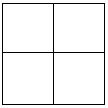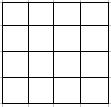You probably do it everyday when you fold a handkerchief into your pocket. So what's new? Ok. Now let's alter the question a little bit. Can you divide a square into smaller squares, so that no two squares are of the same size? Now, that's tricky, isn't it? A. J. W. Duijvestijn was the first mathematician to show that this is possible. Here's a squared square discovered by Duijvestijn. It shows a 112 x 112 square divided into 21 distinct squares.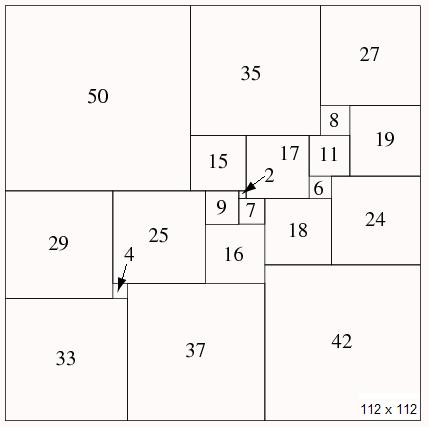Dividing a square into smaller squares is called "squaring a square". In broader terms, it is also referred to as "tiling of a square". If each of the smaller squares is of different size, it is called a "perfect simple squared square". The number of smaller squares used, is called the order of the squaring. Its not a "simple" squared square if a subset of squares group together to form a rectangle. Its not a "perfect" squared sqaure if 2 or more squares have the same size. The square tiling of order 21, discovered by Duijvestijn, is the SMALLEST perfect squared square possible. Now, how do you go about finding out the length of each square in a tiling like that? It's not that difficult. As an example, let's see the 5-order tiling of the square below. Though such a 5-order tiling is not possible, since the smallest order is 21, the example will help us understand the process of finding the lengths easily.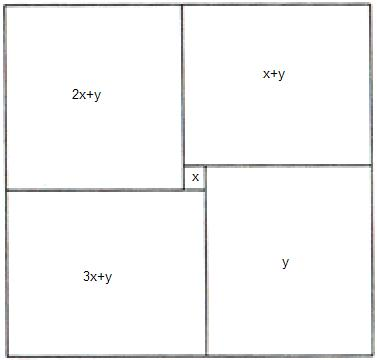We start with assigning lengths x & y to adjacent squares as shown above. Using these 2 values, we can easily calculate the lengths of all the remaining squares in terms of x & y. After we complete this process, all sides of the outer most square can be expressed in terms of x & y. Since the sides of the square are of equal length, we can equate these expressions. What we end up with is the value of y expressed as a multiple of x (eg: y = 5x). Depending on the order of your squaring, you may have to use 3 variables x,y,z instead of just x & y. Nevertheless, you always end up finding all variables as a multiple of x. (eg: y = 5x , z = 7x, etc.) Once we find all variables in terms of x, our job is done. Just set x to any value, say 1. We can then find values of all other variables, as all of them are a multiple of x. At the beginning of this example, we mentioned that such a 5-order tiling is not possible. Like to know why? Just follow the process described above for the picture shown in the example, and try to find the value of x or y. You will know the answer! Interesting? Now let's ask ourselves another question - Is it possible to divide a cube into smaller cubes, no two cubes having the same size? Looking at the example above, we are tempted to assume that we can. However, it is proved to be impossible! Here's a simple explanation why. Every face of a cubed cube is a squared square. Consider one face of such a cube where the smallest square on the face is of side s. Also, the smallest square in a squared square can not lie on the edge of the square, as seen in the Duijvestijn's squared square.Now lets take a peek "inside" the box.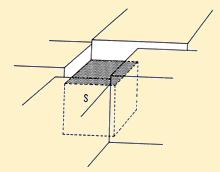Since s is the smallest cube lying on the face of the original cube, the other cubes beside s are "towering" above s. Which means there is a "gap" to be filled above s, walled by the larger cubes beside it. This implies, we need cube(s) smaller than s to fill that gap. Once we try to fill that gap with smaller cubes, we come across the same situation that we came across with cube s. This can continue indefinitely and we can never reach the end point. In other words, we would require INFINITE cubes, to achieve a perfect cubing. Hence,cubing a cube is impossible! (This method of proving a statement is also known as the method of "infinite descent".)
 Quick Comment : * Name Email ( Your email id is safe with us! ) * Comments * Type the number of characters in the word "HUMAN"